Courses
Courses for Kids
Free study material
Offline Centres
More

# NCERT Solutions for Class 9 Science Chapter 11 - Work And EnergyLast updated date: 29th Nov 2023
Total views: 473.4k
Views today: 6.73k

## NCERT Solutions for Class 9 Science Chapter 11 - Work and Energy - Free PDF Download

The class 9 chapter 11 science is now made easier for the students with the comprehensive NCERT Class 9 science solutions chapter 11 PDF. Every topic and subtopic is meticulously elaborated for students to understand clearly. You can now find the PDFs of these explanations on the Vedantu website. These solutions are the one-stop guide to preparing for your exams with ease. Now, refer to them to get in-depth knowledge on essential topics of Work and Energy to ace your exams with ease. Vedantu is a platform that provides free NCERT Solutions and other study materials for students. Maths Students who are looking for better solutions, they can download Class 9 Maths NCERT Solutions to help you to revise complete syllabus and score more marks in your examinations.

Watch videos on
NCERT Solutions for Class 9 Science Chapter 11 - Work And EnergyWork Energy and Power in One-Shot | CBSE Class 9 Physics | Science Chapter 11 | NCERT Solutions
Vedantu 9&10
SubscribeShare
14.3K likes
333.6K Views
3 years ago
Play Quiz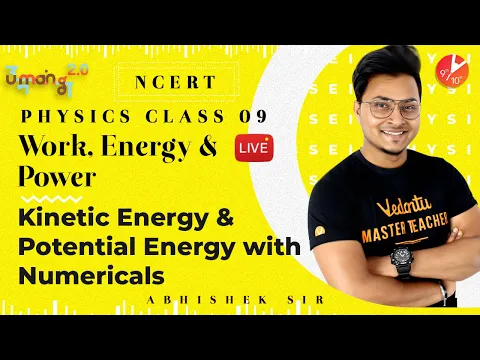Work Energy and Power L2 | Kinetic Energy | CBSE Class 9 Science NCERT |Umang Vedantu Class 9 and 10
Vedantu 9&10
9.7K likes
231.3K Views
3 years ago
Play Quiz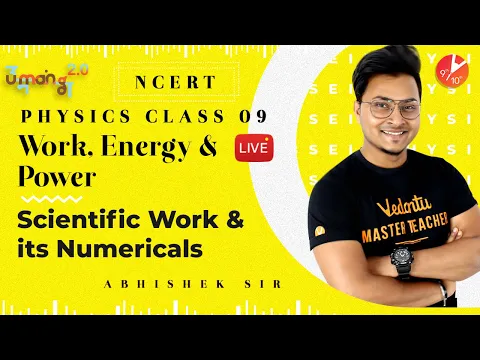Work Energy and Power L1 | Scientific Work and Its Numericals | CBSE Class 9 Science NCERT | Vedantu
Vedantu 9&10
15.7K likes
363.9K Views
3 years ago
Play Quiz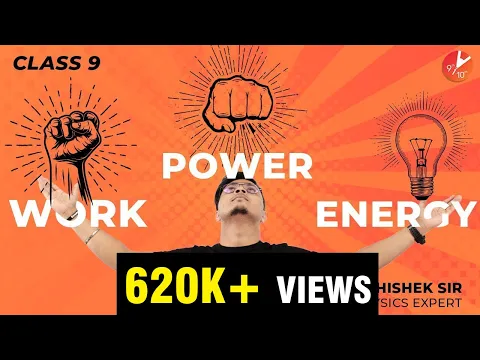Work Energy and Power In 30 Min | CBSE Class 9 Science | Physics | Vedantu Class 9
Vedantu 9&10
38.6K likes
846K Views
4 years ago
Play Quiz

## Access NCERT Answers for Class 9 Science Chapter 11 – Work and energy

### Intext Exercise 1

1. A force of 7 N acts on an object. The displacement is, say 8 m, in the direction of the force (Fig. 11.3). Let us take it that the force acts on the object through displacement. What is the work done in this case?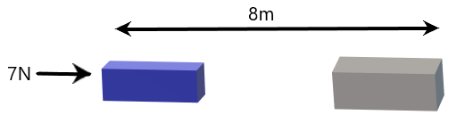Ans: In the above question it is given that:

Force is $F=7N$.

Displacement is $S=8m$

Work done is given by the formula:

$\text{Work done = Force }\!\!\times\!\!\text{ Displacement}$

$W=F\times S=7\times 8=56Nm$

Hence, the work done in this case is $56J$.

### Intext Exercise 2

1. When do we say that work is done?

Ans: Work is said to be done if the following two conditions are satisfied:

1. The force must act on the object

2. The object should be displaced.

2. Write an expression for the work done when a force is acting on an object in the direction of its displacement.

Ans: Consider a constant force $F$ acting on an object. If the object is displaced by a distance $s$ in the direction of the force (Fig. 11.1) and $W$ is the work done. Hence work done is equal to the product of the force and displacement.

$\text{Work done = Force }\!\!\times\!\!\text{ Displacement}$

$\Rightarrow W=F\times S$

3. Define 1 J of work.

Ans: $1J$ of work is the amount of work done on an object when a force of $1N$ displaces it by $1m$ along the line of action of the force.

4. A pair of bullocks exerts a force of 140 N on a plough. The field being ploughed is 15m long. How much work is done in ploughing the length of the field?

Ans: In the above question it is given that:

Applied force is $F=140N$.

Displacement is $s=15m$.

We know that

$\text{Work done = Force }\!\!\times\!\!\text{ Displacement}$

$\Rightarrow W=F\times S=140\times 15=2100J$

Therefore, work done in ploughing the length of the field is $2100J$.

### Intext Exercise 3

1. What is the kinetic energy of an object?

Ans: The energy possessed by an object due to its motion is called kinetic energy.

2. Write an expression for the kinetic energy of an object.

Ans: Consider a body of mass $'m'$ to be moving with a velocity $'v'$. Hence its kinetic energy ${{E}_{k}}$ is given by:

${{E}_{k}}=\frac{1}{2}m{{v}^{2}}$

The SI unit of kinetic energy is the Joule $\left( J \right)$.

3. The kinetic energy of an object of mass m moving with a velocity of 5 m/s is 25J.What will be its kinetic energy when its velocity is doubled? What will be its kinetic energy when its velocity is increased three times?

Ans: In the above question it is given that:

Velocity of the object is $5m/s$.

Kinetic energy of the object is ${{E}_{k}}=25J$.

Mass of the object is $m$.

We know that,

${{E}_{k}}=\frac{1}{2}m{{v}^{2}}$

Case 1: When the velocity of an object is doubled,

$v=10m/s$

Kinetic Energy is given by:

${{E}_{k}}=\frac{1}{2}m{{v}^{2}}$

${{E}_{k}}\propto {{v}^{2}}$

Hence, when $v=10m/s$

${{E}_{k}}=25\times 4=100J$

The kinetic energy becomes 4 times its initial value.

Case 2: If velocity is increased three times, then its kinetic energy will be nine times its original value, as ${{E}_{k}}\propto {{v}^{2}}$.

Hence, kinetic energy will be:

${{E}_{k}}=25\times 9=225J$

Thus, kinetic energy becomes nine times its initial value.

### Intext Exercise 4

1. What is power?

Ans: Power is defined as the rate of doing work or the rate of transfer of energy. If W is the amount of work done in time t, then power is given by:$\text{Pow}er=\frac{Work\text{ }done}{Time}=\frac{Energy}{Time}$

$P=\frac{W}{T}$

The S.I. unit power is watt $\left( W \right)$.

2. Define 1 watt of power.

Ans: The power of an agent, which does work at the rate of 1 joule per second is defined as $1$ watt. It is also said that power is $1W$ when the rate of consumption of energy is $1J/s$.

$1W=\frac{1J}{1s}$

3. A lamp consumes 1000 J of electrical energy in 10 s. What is its power?

Ans: In the above question it is given that:

Energy consumed is $E=1000J$

Time $T=10s$

$\text{Pow}er=\frac{Work\text{ }done}{Time}=\frac{Energy}{Time}$

$\Rightarrow Power=\frac{1000}{10}=100W$

Hence, the power generated is$100W$.

4. Define average power.

Ans: Average power is defined as the power obtained by dividing the total amount of work done in the total time taken to do this work.$\text{Average power}=\frac{\text{Total work done}}{\text{total time taken}}$

### NCERT Exercise

1. Look at the activities listed below. Reason out whether or not work is done in the light of your understanding of the term ‘work’.

• Suma is swimming in a pond.

• A donkey is carrying a load on its back.

• A wind mill is lifting water from a well.

• A green plant is carrying out photosynthesis.

• An engine is pulling a train.

• Food grains are getting dried in the sun.

• A sailboat is moving due to wind energy.

Ans: According to the definition, work is done whenever the following two conditions are satisfied:

1. A force acts on the body.

2. There is a displacement of the body by the application of force in or opposite to the direction of force.

Therefore,

• In this case of swimming, Suma applies a force to push the water backwards. This allows Suma to swim in the forward direction by the forward response of water. Hence, the force causes a displacement. Thus, while swimming work is done by Suma.

• In this case the donkey applies a force in the upward direction while carrying a load. But the displacement of the load is in the forward direction. As the displacement is perpendicular to force, the work done is zero.

• In this case work is done by the windmill in lifting water from the well as a windmill works against the gravitational force to lift water.

• In this case there is no displacement of the leaves of the plant. Hence, the work done is zero.

• In this case an engine applies force to pull the train. This makes the train move in the direction of the applied force. Thus, there is a displacement in the train in the same direction. Hence the work is done by the engine on the train.

• In this case during the process of food grains getting dried in the sun, no work is done as food grains do not displace in the presence of solar energy.

• In this case wind energy applies a force on the sailboat to push it in forward direction. Therefore, the displacement of the boat is along the direction of the force. Hence, the work is done by wind on the boat.

2. An object thrown at a certain angle to the ground moves in a curved path and falls back to the ground. The initial and the final points of the path of the object lie on the same horizontal line. What is the work done by the force of gravity on the object?

Ans: In the above question the object thrown at a certain angle to the ground moves in a curved path and falls back to the ground. The initial and the final points of the path of the object lie on the same horizontal line.

Work done by the force of gravity on an object depends only on vertical displacement. Vertical displacement is given by the difference in the initial and final positions (height) of the object, which is zero.

Work done by gravity is expressed as, $W\text{ }=\text{ }mgh$

Where,

m is the mass of the object,

g is the acceleration due to gravity

h is the vertical displacement, which is zero.

$\Rightarrow W\text{ }=\text{ }mg\left( 0 \right)=0J$

Thus, the work done by gravity on the given object is zero joules.

3. A battery lights a bulb. Describe the energy changes involved in the process.

Ans: Once a bulb is connected to a battery its chemical energy is converted into electrical energy. When the bulb receives this electrical energy, it is converted into light and heat energy. Therefore, the transformation of energy in the given situation can be shown as:$\text{Chemical Energy}\to \text{Electrical Energy}\to \text{Light Energy}\to \text{Heat energy}$

4. Certain force acting on a 20 kg mass changes its velocity from $5m/s$ to $2m/s$. Calculate the work done by the force.

Ans: Change in kinetic energy is defined as the work done.

It is given that a 20 kg mass changes its velocity from $5m/s$ to $2m/s$.

Kinetic energy is given by the expression,

$\left( {{E}_{k}} \right)v=\frac{1}{2}m{{v}^{2}}$

Where,

${{E}_{k}}=$ Kinetic energy of the object moving with a velocity, v

$m=$ Mass of the object

Kinetic energy when the object was moving with a velocity $5m/s$,${{\left( {{E}_{k}} \right)}_{5}}=\frac{1}{2}\times 20\times {{\left( 5 \right)}^{2}}=250J$

Kinetic energy when the object was moving with a velocity $2m/s$,${{\left( {{E}_{k}} \right)}_{2}}=\frac{1}{2}\times 20\times {{\left( 2 \right)}^{2}}=40J$

Therefore, work done by force $=40-250=-210J$

Here, the negative sign shows that the force is acting in the opposite direction of the motion of the object.

5. A mass of 10 kg is at a point A on a table. It is moved to a point B. If the line joining A and B is horizontal, what is the work done on the object by the gravitational force? Explain your answer.

Ans: Work done by gravity depends only on the vertical displacement of the body. It does not depend upon the path of the body. Hence, work done by gravity is given by:

$W\text{ }=\text{ }mgh$

Where,

Vertical displacement, $h=0$

$\Rightarrow W\text{ }=\text{ }mg\left( 0 \right)=0J$

Thus, the work done by gravity on the object is zero joules.

6. The potential energy of a freely falling object decreases progressively. Does this violate the law of conservation of energy? Why?

Ans: When the body drops from a height, its potential energy changes into kinetic energy progressively. A decrease in the potential energy is equivalent to an increase in the kinetic energy of the body.

During this process, the total mechanical energy of the body is conserved. Therefore, the law of conservation of energy is not violated.

7. What are the various energy transformations that occur when you are riding a bicycle?

Ans: When we ride a bicycle, our muscular energy is transferred into the bicycle's heat energy and kinetic energy. Heat energy heats the body and kinetic energy provides a velocity to the bicycle. The transformation can be shown as:$Muscular\text{ Energy}\to Kinetic\text{ Energy}\to \text{Heat energy}$

The total energy is conserved in the entire transformation of energies.

8. Does the transfer of energy take place when you push a huge rock with all your might and fail to move it? Where is the energy you spend going?

Ans: When we push a huge rock there is no transfer of energy because there is no transfer of muscular energy to the stationary rock. Instead muscular energy is transferred into heat energy, which causes our body to become hot.

9. A certain household has consumed 250 units of energy during a month. How much energy is this in joules?

Ans: We know that $1$ unit of energy is equal to $1$ kilowatt hour (kWh).$1\text{ }unit\text{ }=\text{ }1\text{ }kWh$

Therefore,

$1kWh=3.6\times {{10}^{6}}J$

Hence, $250$ units of energy $=250\times 3.6\times {{10}^{6}}=9\times {{10}^{8}}J$.

10. An object of mass 40 kg is raised to a height of 5 m above the ground. What is its potential energy? If the object is allowed to fall, find its kinetic energy when it is half-way down.

Ans: In the above question it is given that:

$h\text{ }=\text{ }Vertical\text{ }displacement\text{ }=\text{ }5\text{ }m$

$m\text{ }=\text{ }Mass\text{ }of\text{ }the\text{ }object\text{ }=\text{ }40\text{ }kg$$g\text{ }=\text{ }Acceleration\text{ }due\text{ }to\text{ }gravity\text{ }=\text{ }9.8\text{ }m/{{s}^{2}}$

Gravitational potential energy is given by the expression,

$W\text{ }=\text{ }mgh$

$\Rightarrow W\text{ }=\text{ }40\times 5\times 9.8\text{ }=\text{ }1960\text{ }J$

At half-way down, the potential energy of the object will reduce to half i.e.,  $\frac{1960}{2}=980J$.

At this point, the object has potential energy equal to kinetic energy. This is due to the law of conservation of energy. Hence, half-way down, the kinetic energy of the object will be $980J$.

11. What is the work done by the force of gravity on a satellite moving round the earth? Justify your answer.

Ans: When the direction of force is perpendicular to displacement, the work done is zero. If a satellite moves around the Earth the direction of force of gravity on the satellite is perpendicular to its displacement. Therefore, the work done on the satellite by the Earth is zero.

12. Can there be displacement of an object in the absence of any force acting on it? Think. Discuss this question with your friends and teacher.

Ans: Yes, there be displacement of an object in the absence of any force acting on it by moving with uniform velocity. Suppose an object is moving with constant velocity, then net force acting on it is zero. Hence, there can be a displacement without a force.

13. A person holds a bundle of hay over his head for 30 minutes and gets tired. Has he done some work or not? Justify your answer.

Ans: If a person holds a bundle of hay over his head, gravitational force is acting on the hay downwards. But, there is no displacement in the bundle of hay in the direction of force. Hence, no work is done.

14. An electric heater is rated 1500 W. How much energy does it use in 10 hours?

Ans: Energy consumed by an electric heater is given by the expression,

$P=\frac{W}{T}$

Where,

$P\text{ }=\text{ }1500\text{ }W\text{ }=\text{ }1.5\text{ }kW$

$T\text{ }=\text{ }10\text{ }hrs$

We know that,

$Work\text{ }done\text{ }=\text{ }Energy\text{ }consumed\text{ }by\text{ }the\text{ }heater$

Hence, $energy\text{ }consumed\text{ }=\text{ }Power\text{ }\times \text{ }Time=1.5\times 10=15kWh$= 1.5 × 10 = 15 kWh.

15. Illustrate the law of conservation of energy by discussing the energy changes which occur when we draw a pendulum bob to one side and allow it to oscillate. Why does the bob eventually come to rest? What happens to its energy eventually? Is it a violation of the law of conservation of energy?

Ans: According to the “law of conservation of energy”: Energy can be neither created nor destroyed. It can only be converted from one form to another. Consider the case of an oscillating pendulum.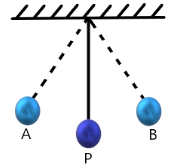When a pendulum moves from its mean position P to either of its extreme positions A or B, it rises through a height h above the mean level P. At the extreme point, bob comes to rest and the kinetic energy of the bob is transformed into potential energy.

As it moves towards point P, its potential energy decreases progressively. Simultaneously, the kinetic energy increases.

As the bob reaches point P, its potential energy is converted to kinetic energy. At this point bob possesses only kinetic energy. This process is repeated as long as the pendulum oscillates. The bob does not oscillate forever. It comes to rest due to some friction in the air.

The pendulum loses energy overcoming this friction. After all the energy is lost, it comes to rest. The law of conservation of energy holds here as the energy lost by the pendulum to overcome friction is gained by its surroundings. Hence, the total energy of the pendulum and the surrounding system is conserved.

16. An object of mass m is moving with a constant velocity, v. How much work should be done on the object in order to bring the object to rest?

Ans: When a body is in motion it possesses kinetic energy. Kinetic energy of an object of mass, m moving with a velocity, v is given by the expression,

${{E}_{k}}=\frac{1}{2}m{{v}^{2}}$

Therefore, $\frac{1}{2}m{{v}^{2}}$ amount of work is required to be done on the object to bring the object to rest.

17. Calculate the work required to be done to stop a car of 1500 kg moving at a velocity of 60 km/h?

Ans: In the above question it is given that:

Mass of car, $m\text{ }=\text{ }1500\text{ }kg$

Velocity of car, $v\text{ }=\text{ }60\text{ }km/h\text{ }=60\times \frac{5}{18}m/s$

Kinetic energy is given by:

${{E}_{k}}=\frac{1}{2}m{{v}^{2}}$

$\Rightarrow {{E}_{k}}=\frac{1}{2}\left( 1500 \right){{\left( 60\times \frac{5}{18} \right)}^{2}}=20.8\times {{10}^{4}}J$

Hence, $20.8\times {{10}^{4}}J$ needs to be done to stop the car.

18. In each of the following a force, F is acting on an object of mass, m. The direction of displacement is from west to east shown by the longer arrow. Observe the diagrams carefully and state whether the work done by the force is negative, positive or zero.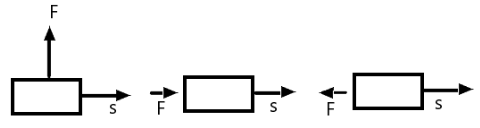Ans: Following cases are explained below:

Case I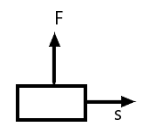Here the direction of force acting on the block is perpendicular to the displacement.

Hence, work done by force on the block will be zero.

Case II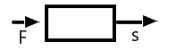Here the direction of force acting on the block is in the direction of displacement. So, work done by force on the block will be positive.

Case III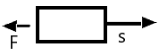Here the direction of force acting on the block is opposite to the direction of displacement. Hence, work done by force on the block will be negative.

19. Soni says that the acceleration in an object could be zero even when several forces are acting on it. Do you agree with her? Why?

Ans: Acceleration of an object will be zero when net force acting on an object will be zero. Net force can be zero even when there are multiple forces acting on the body. For a uniformly moving object, the net force acting on the object is zero. Hence, the acceleration of the object is zero. Hence, Soni is right.

20. Find the energy in kWh consumed in 10 hours by four devices of power 500 W each.

Ans: In the above question it is given that:

$P\text{ }=\text{ }500\text{ }W\text{ }=\text{ }0.50\text{ }kW$

$T\text{ }=\text{ }10\text{ }hrs$

Energy consumed by an electric device can be obtained with the help of the expression given below:

$P=\frac{W}{T}$

Where,

$Work\text{ }done\text{ }=\text{ }Energy\text{ }consumed\text{ }by\text{ }the\text{ }device$$Energy\text{ }consumed\text{ }by\text{ }each\text{ }device\text{ }=\text{ }Power\text{ }\times \text{ }Time$$\text{Work done}=\text{ }0.50\text{ }\times \text{ }10\text{ }=\text{ }5\text{ }kWh$

Hence, the energy consumed by four equal rating devices in $10\text{ }hrs$ will be $4\times 5kWh=20kWh$

21. A freely falling object eventually stops on reaching the ground. What happens to its kinetic energy?

Ans: Once the object hits the hard ground, the kinetic energy gets converted into heat energy and sound energy. Further, entire energy is lost to the environment. This energy can also deform the ground with respect to the nature of the ground and the amount of kinetic energy possessed by the object.

You can opt for Chapter 11 - Work and Energy NCERT Solutions for Class 9 Science PDF for Upcoming Exams and also You can Find the Solutions of All the Maths Chapters below.

NCERT Solutions for Class 9 Science

Along with this, students can also view additional study materials provided by Vedantu, for Class 9

To get hands-on an elaborate presentation of Class 9 work and energy solutions, go through the free PDFs offered on the official webpage of Vedantu. Grasp all essential topics for preparing well for your exam.

## List of Topics Covered Under NCERT Solutions for Class 9 Science Chapter 11 - Work and Energy

1. Work

• The scientific conception of Work

• Work done by a constant force

1. Energy

• Forms of energy

• Kinetic energy

• Potential energy

• Potential energy of an object at a height

• Law of conservation of energy

1. Rate of Doing Work

• Commercial unit of Work

### A Glance About The Class 9 Science Chapter 11 - Work and Energy

• The work done is the product of force acting on an object and displacement of the object

• The capacity of an object to do work is called Energy

• The energy is of two types, kinetic and potential energy

• Chemical energy, Mechanical energy, Light energy..etc are the forms of energy.

• An object of mass that moves with constant velocity is called Kinetic energy

• The energy which is transferred and stored to the object, while work is done is called Potential energy.

## NCERT Solutions for Class 9 Science Chapter 10 - Work and Energy

### 11.1 Work

The NCERT solutions for class 9 science chapter 11 involve brief explanations of work and energy. They offer insights into the very concept of work and how ‘work’ is defined in our everyday life. Discover some prime examples through the below-mentioned sub-topics to know more about work.

### 11.1.1 Not Much ‘Work’ in Spite of Working Hard

NCERT Class 9 science chapter 11 solutions give students insights into distinguishing examples of work. This is explained by talking about the core subject, Kamali. She is keen on preparing for her exams. She is dedicated to following her time table and studying for exams whenever feasible. She organizes, analyses, and maps her thoughts, thereby collecting relevant study materials for studying.

Thus, all these activities that Kamali performs require a sufficient amount of energy. In standard parlance, Kamali is working hard. In a nutshell, the definition of work varies from individual to individual. Activities like talking to friends, going out with your family, playing your favourite game, or watching a movie fall under the ‘not much work despite working hard’ category.

### 11.1.2 Scientific Conception of Work

Activities where the subject and object both have an impact after work is being done, in general, is nothing hit the scientific conception of work. Work and Energy Class 9 NCERT Solutions deal with different situations that fall under the scientific conception of work.

From a scientific perspective, work can be defined as pushing a pebble lying down on a surface, lifting a book through a respective height, and so on. Thus, for work two be done, two conditions need to be satisfied. These include- the action of force on an object and the displacement of the item.

### 11.1.3 Work Done By a Constant Force

Ever wondered how work is defined by science? One can easily understand this by a simple demonstration as follows. Take a situation wherein the force is acting in the very direction of displacement.

Now, consider a constant force F acting on a specific object. Let this object be displayed by a particular distance S in the exact direction of the force. Here:

Work Done = Force x Displacement

W = Fs

Dive into the ncert solutions for class 9 chapter 11 to know more.

### 11.2 Energy

We must all understand by now that energy is a necessity for life itself. The need for energy seems to increase at an exceeding rate. However, the question lies, where does energy come from?

Well, the sun constitutes the largest natural source of energy for living creatures. Several energy sources are primarily extracted from the sun. Additionally, we can also derive energy from nuclei of atoms, tides, and the interior of the earth.

### 11.2.1 Forms of Energy

Our world offers a myriad of forms of energy. These include- potential energy + kinetic energy (mechanical energy), light energy, chemical energy, electrical energy, and heat energy.

### 11.2.2 Kinetic Energy

The kinetic energy of a certain object is nothing but the energy that it consists of because of its motion. It is known as the work required to accelerate the respective body of a mass from rest to its actual velocity.

### 11.2.3 Potential Energy

The energy possessed by the respective body mainly due to the change of shape or position is known as potential energy.

### 11.2.4 Potential Energy of An Object At A Height

A particular object enhanced its energy after being raised by a height. This happens because work is being done on it in opposition to gravity while being raised. This energy available in a relevant object is known as gravitational potential energy.

### 11.2.5 Are Various Energy Forms Inconvertible?

Is energy conversion from one form to another possible? Go through NCERT Solutions for Class 9 Science Chapter 11 to understand how energy conversion to different forms occurs.

11.2.6 Law of Conservation of Energy

The law of conservation of energy states that energy is only transformed from one form to another. It cannot be created, not destroyed. The sum total of energy before and after the transformation is always constant.

### 11.3 Rate of Doing Work

Power is primarily defined as the overall rate of doing work. The SI unit of power is defined as a watt.

1W = 1 J/s

### 11.3.1 Commercial Unit of Energy

Joule is an extremely small unit and is thus not reliable to define definite and vast quantities of energy. This is why a bigger unit called kilowatt hour (kW h) is used. The energy accessed in about an hour at the rate of 1kW is known as 1 kW h.

### Key Features of NCERT Solutions for Class 9 Science Chapter 11

Key Features of NCERT Solutions, These solutions are designed to help students achieve proficiency in their studies. They are crafted by experienced educators who excel in teaching class 9 Science. Some of the features include:

• Comprehensive explanations for each exercise and questions, promoting a deeper understanding of the subject.

• Clear and structured presentation for easy comprehension.

• Accurate answers aligned with the curriculum, boosting students' confidence in their knowledge.

• Visual aids like diagrams and illustrations to simplify complex concepts.

• Additional tips and insights to enhance students' performance.

• Chapter summaries for quick revision.

## Conclusion

The NCERT Solutions for Class 9 chapter 11, Science - Word and Energy, provided by Vedantu, is a valuable tool for Class 9 students. It helps introduce Science concepts in an accessible manner. The provided solutions and explanations simplify complex ideas, making it easier for Class 9 Students to understand the material. By using Vedantu's resources, Students can develop a deeper understanding of NCERT concepts. These solutions are a helpful aid for class 9 students, empowering them to excel in their studies and develop a genuine appreciation for Work and Energy.

## FAQs on NCERT Solutions for Class 9 Science Chapter 11 - Work And Energy

1. How Many Exercises Does this Chapter Include?

Work and energy is a vast chapter that includes about 5 exercises in total. These are mentioned below.

• Exercise 11.1 - 1 Question on work and power.

• Exercise 11.2 - 4 questions on energy and units of energy.

• Exercise 11.3 - 3 questions on different forms of energy.

• Exercise 11.4 - 4 questions on kinetic energy and its expression.

• Exercise 11.5 - 21 questions on potential energy and its expression and conservation of energy.

2. Why Should you Rely on Vedantu NCERT Solutions?

NCERT solutions in class 9 Chapter 11 by Vedantu aim to help students get definite clarity on what they should focus on while preparing for their exams. Along with brief summaries on every topic and subtopic, you will also have access to different question banks that you can refer to. Further, all the questions and answers and explanations are precise, easy to understand, and reliable for quick preparation of exams. With the right study material, you can take the benefit of the doubt and gain essential knowledge on the concepts of work and energy.

3. What is the kinetic energy of an object in Chapter 11 of NCERT Solutions for Class 9 Science?

The Kinetic Energy of a particular object is the energy that it has  because of its motion. It is known as the work required to accelerate the respective body of a given mass from rest to its actual velocity. Thus, any object in motion always has kinetic energy. For instance, a person walking or running, a thrown baseball, a crumb falling from a tabletop are all examples of Kinetic Energy. To know more about it, visit Vedantu website or Vedantu Mobile app. The solutions provided by Vedantu are free of cost.

4. What is work according to Chapter 11 of Class 9 Science NCERT?

Work done is as the product of two components- force (F) acting in the direction of the displacement and the magnitude of displacement (s) of the object. Thus, the two conditions required for work to be done are the action of force on an object and the displacement of the object. The formula for work done is: Work Done = Force x Displacement, W = Fs.  It is a scalar quantity (because it has only magnitude and no direction) and it is measured in Joules (J).

5. What is energy according to Chapter 11 of Class 9 Science NCERT?

A body's Energy is defined, in Physics, as the capacity of that body to perform work. Energy has only magnitude and no direction i.e., it is a scalar quantity and is measured in Joules (J). The Sun constitutes the largest natural source of energy for all living creatures. Some other energy sources are nuclei of atoms, tides, and the interior of the Earth. There are several types of energy, such as Potential Energy, Kinetic Energy, Mechanical Energy, Light Energy, Chemical Energy, Electrical Energy, and Heat Energy.

6. What is the formula of Energy according to Chapter 11 of NCERT Solutions for Class 9 Science?

A body's Energy, in Physics, is defined as the capacity of that body to perform work. Energy’s SI unit is Joules. It is scalar in nature as it has only magnitude and no direction. The formula for Potential Energy, Ep, of an object is equivalent to the work done over an object of mass 'm' to raise it by a height 'h' when 'g' is the acceleration due to gravity. Therefore, Ep = mgh.

7. What is Work and Energy in Physics of Class 9?

In physics, work is defined as the sum of two factors: the force acting in the direction of displacement (F) and the amount of the object's displacement (s). The capacity of a body to produce work is defined as its energy in physics. Both Work and Energy are scalar quantities that are measured in Joules (J).